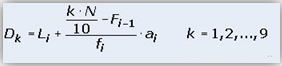# Percentile Rank, Decile, Ordinal Rank Calculator#Percentile and Ordinal Rank Calculator

An online percentile calculator with ordinal rank.

Percentile Rank Formula

Rank = (P / 100) * N

Where,

P is Percentile,

N is Number of items

Decile FormulaWhere,

L is lower boundary

F is cummulative frequency

f is frequency

N is total frequency

k = nth decile, where n = 1, 2, 3, 4, 5, 6, 7, 8, and 9## How to find percentile and ordinal rank ?

Example Question: Find out where the 25th percentile is in the below list.

ScoreRank
3001
3302
4303
5304
5605
6706
6807
7208

Step 1:Arrange the data in ascending order

30, 33, 43, 53, 56, 67, 68, 72

Step 2:Calculate what rank is at the 25th percentile by using the following formula

Rank = P / 100 * (N)

Where P is Percentile = 25, N is Number of items = 8

Rank = 25 / 100 * (8) = 0.25 * 8 = 2.

The 25th percentile is the average of the values in the 1th and 2th positions (33 and 43 respectively).

The 25th percentile is (33 + 43) / 2 = 38

In this case, the 25th percentile score is 38, which makes more sense as its in the middle of 43 and 33.